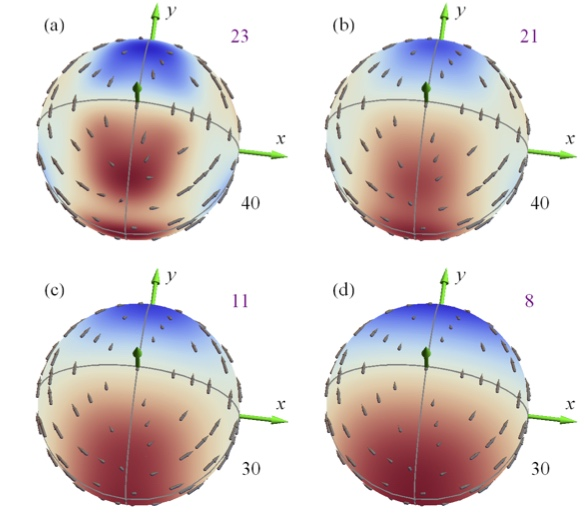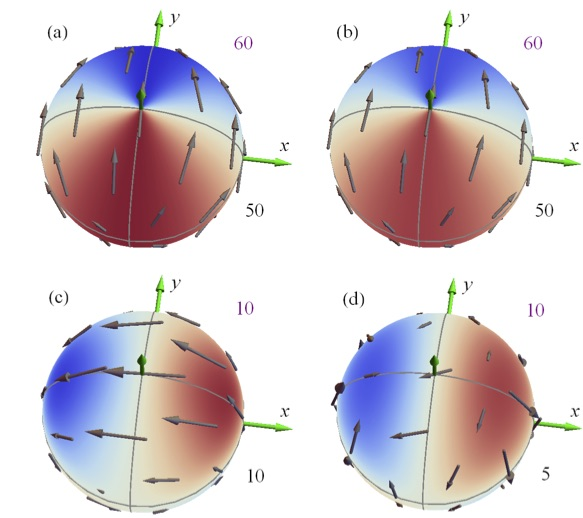Examples

# Spin-orbit Torques in CoPt Multilayers

We have demonstrated the feasibility of calculating the spin-orbit torques in layered systems within density-functional theory, augmented by an Anderson model to treat disorder. Terms beyond the usual damping-like and ﬁeld-like torques were found. While the torques that contribute to damping are almost entirely due to spin-orbit coupling on the Pt atoms, the field-like torque does not require it.

In this paper, the spin-orbit torque in a disordered Co/Pt bilayer is calculated using a ﬁrst-principles non- equilibrium Green’s function formalism. The torque is formally split in the Fermi-sea and Fermi- surface contributions. The Fermi-sea term is sizeable at low temperatures, but the Fermi-surface term dominates at room temperature.

A symmetry analysis of the total effect can be divided into terms related to phenomenological theories, in particular a field-like term $(\mathbf{z} \times \mathbf{E})\times \mathbf{m}$, which is odd with respect to the magnetization $\mathbf{m}$, and a damping-like term $\mathbf{m}\times[(\mathbf{z} \times \mathbf{E})\times \mathbf{m}]$, which is even in $\mathbf{m}$.

In addition to the usual damping-like and ﬁeld-like terms, the odd torque contains a sizeable planar Hall-like term $(\mathbf{m} \cdot \mathbf{E})\mathbf{m} \times (\mathbf{z} \times \mathbf{m})$, which contributes to damping. This term is is consistent with ferromagnetic resonance measurements.

This tour-de-force calculation illustrates some recently developed capabilities in the layer Green’s function program.

The dissipative part of the spin-orbit torque is almost entirely due to spin-orbit coupling on the heavy Pt site. However the field-like term does not require a heavy atom with large spin-orbit coupling.The figure above shows the Fermi sea contribution to the torque at different temperatures. The figure below shows the Fermi surface contribution to the torque for differing degrees of Anderson disorder.This work has been submitted to Physical Review Letters.

PAPERS · NONEQUILIBRIUM GREEN'S FUNCTIONS